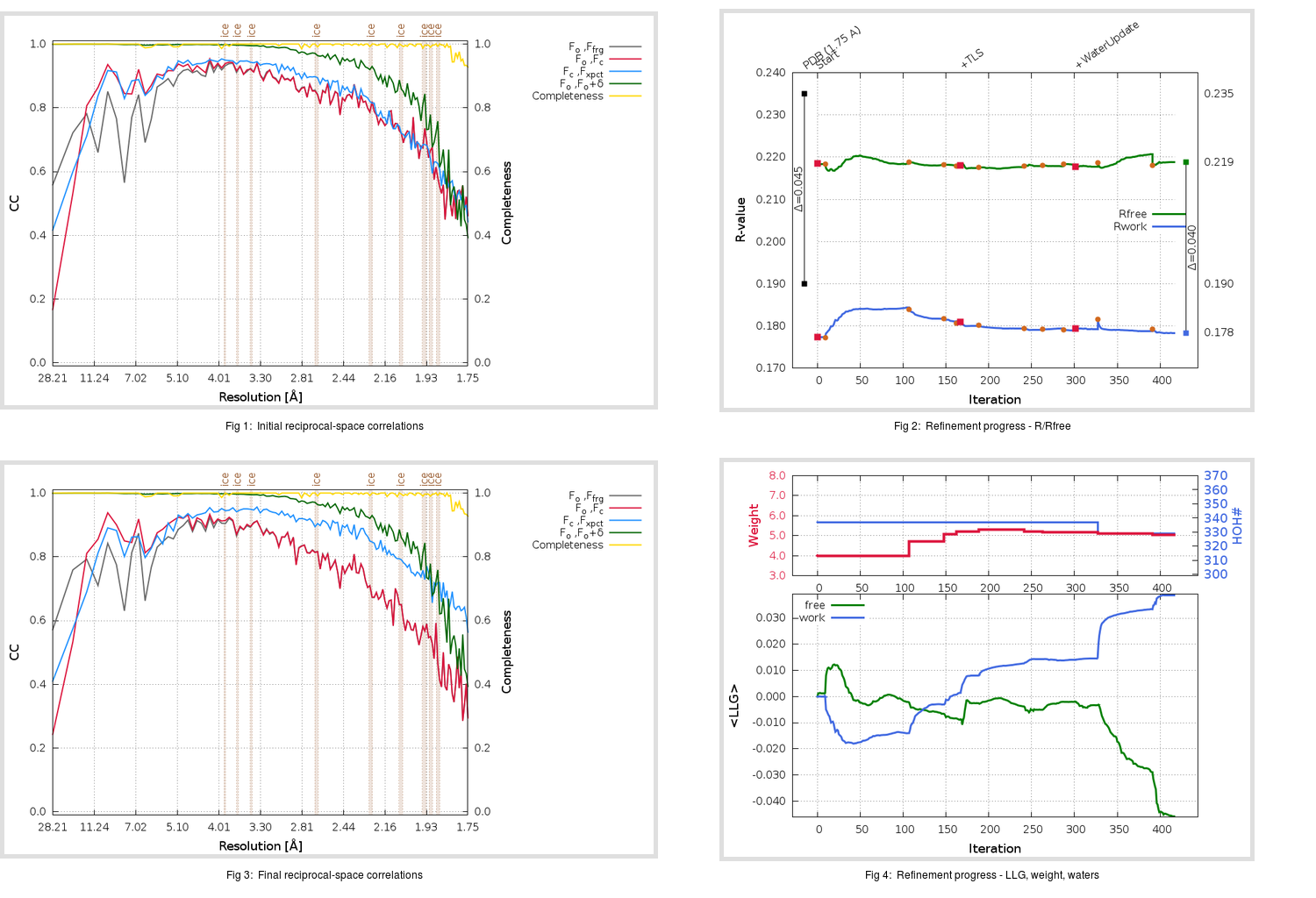Content:

## Deposited

` `
 Date deposited Date data collection Resolution R, Rfree 20200315 20200305 1.75 0.1880 0.2350

Molprobity (CCP4 7.0 version) summary:

```Ramachandran outliers =   0.33 %
favored =  97.02 %
Rotamer outliers      =   1.14 %
C-beta deviations     =     0
Clashscore            =   4.86
RMS(bonds)            =   0.0135
RMS(angles)           =   1.81
MolProbity score      =   1.47
Resolution            =   1.75
R-work                =   0.1880
R-free                =   0.2350
```

```Number of waters      =   337

<B> (all atoms) =   25.61 ( sd =    9.56 ) for       2737 non-hydrogen atoms
<B>   (protein) =   24.21 ( sd =    8.76 ) for       2370 non-hydrogen atoms
<B>     (water) =   34.49 ( sd =    9.94 ) for        337 non-hydrogen atoms
<B>    (others) =   37.64 ( sd =    9.73 ) for         30 non-hydrogen atoms

B min/max       (all non-hydrogen atoms) =   13.52 /   73.90
B min/max   (protein non-hydrogen atoms) =   13.52 /   73.90
B min/max     (water non-hydrogen atoms) =   15.00 /   61.92
B min/max     (other non-hydrogen atoms) =   24.12 /   48.60
```

## BUSTER (re-)refinement

` `

Molprobity (CCP4 7.0 version) summary:

```Ramachandran outliers =   0.33 %
favored =  98.01 %
Rotamer outliers      =   0.76 %
C-beta deviations     =     0
Clashscore            =   2.11
RMS(bonds)            =   0.0113
RMS(angles)           =   1.55
MolProbity score      =   0.98
Resolution            =   1.75
R-work                =   0.1784
R-free                =   0.2189
```

```Number of waters      =   329

<B> (all atoms) =   26.81 ( sd =    8.62 ) for       2729 non-hydrogen atoms
<B>   (protein) =   25.27 ( sd =    7.24 ) for       2370 non-hydrogen atoms
<B>     (water) =   37.03 ( sd =   10.27 ) for        329 non-hydrogen atoms
<B>    (others) =   36.30 ( sd =   10.08 ) for         30 non-hydrogen atoms

B min/max       (all non-hydrogen atoms) =   13.60 /   72.85
B min/max   (protein non-hydrogen atoms) =   14.85 /   68.88
B min/max     (water non-hydrogen atoms) =   13.60 /   72.85
B min/max     (other non-hydrogen atoms) =   26.07 /   45.36
```

Refinement progression:Results:

` `
 File Remark 5RFK_aB_refine.01_03_refine.pdb.gz exact refinement commands are in header 5RFK_aB_refine.01_03_refine.mtz.gz including original deposited data and several re-refinement map coefficients 5RFK_aB_refine.01_03_BUSTER_model.cif.gz including any non-standard compound restraints 5RFK_aB_refine.01_03_BUSTER_refln.cif.gz